## Types of Charging

Types of Charging

Charging means gaining or losing electron. Matters can be charged with three ways, charging by friction, charging by contact and charging by induction.

Charging by Friction

When you rub one material to another, they are charged by friction. Material losing electron is positively charged and material gaining electron is negatively charged. Amount of gained and lost electron is equal to each other. In other words, we can say that charges of the system are conserved. When you rub glass rod to a silk, glass lose electron and positively charged and silk gain electron and negatively charged.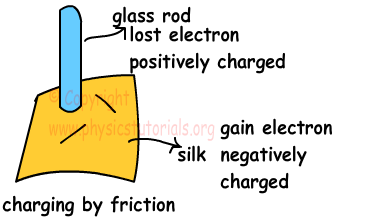Charging by Contact

There are equal numbers of electrons and protons in a neutral matter. If something changes this balance we can say it is charged. Look at the following picture;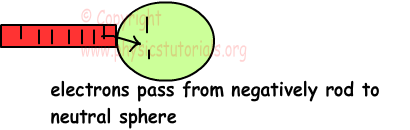In this picture, negatively charged rod touches to the neutral sphere and some of the electrons pass to the sphere. As a result neutral sphere is charged by contact. If the rod is positively charged, then some of the electrons of sphere pass to the rod and when we separate them, sphere becomes positively charged. Picture given below shows the flow of electrons from sphere to the rod.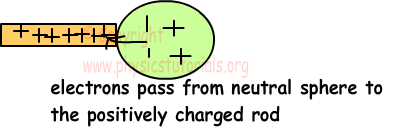1. When charged object touches to a neutral object, they both have same charge.

2. When two charged matter touch each other, total charge of the system is conserved and they share the total charge according to their capacities. If they have same amount of different charges, when we touch one another they become neutral. If the amount of charges is different then, after flow of charge they are both negatively or positively charged. Having opposite charges after contact is impossible.

3. If the touching objects are spheres, they share the total charge according to their radii, because their capacities are directly proportional to their radius. When the spheres are identical then they share total charge equally.

4. We can find the charge per radius by the following equation;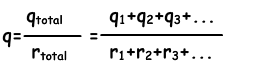Then we use following equation to find the charge per spheres having radii r1 and r2;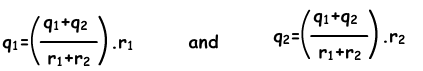Example: Charged spheres having radiuses 3r, 2r and r have different charges. If we touch three spheres to each other, find the final charges of the spheres.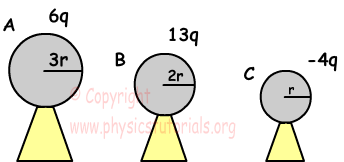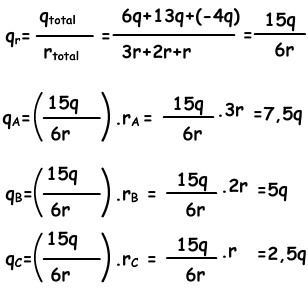Charging by Induction

We can also charge conductors without contact. Examine the given picture, it shows this type of charging.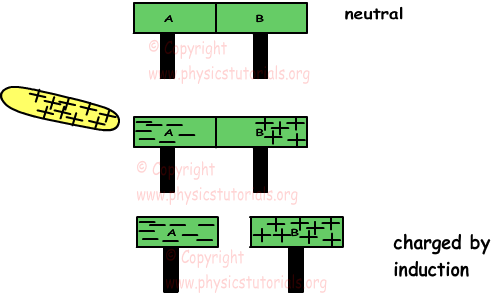A and B conductors are neutral at the beginning. When we put a positively charged plate near them, it attracts the electrons in the conductors. Electrons move to the left part and protons stays. Thus, when we separate plates A and B they are charged by induction, A is negatively charged and B is negatively charged. Be careful, there is no contact, they are charged only by induction.

Electrostatics Exams and Solutions

Author: